## A Rotated Stern-Gerlach Apparatus*

Imagine a Stern-Gerlach apparatus that first separates anatomic beam with a strong B-field gradient in the z-direction. Let's assume the beam has atoms moving in the y-direction. The apparatus blocks two separated beams, leaving only the eigenstate ofwith eigenvalue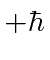. We follow this with an apparatus which separates in the u-direction, which is at an anglefrom the z-direction, but still perpendicular to the direction of travel of the beam, y. What fraction of the (remaining) beam will go into each of the three beams which are split in the u-direction?

We could represent this problem with the following diagram.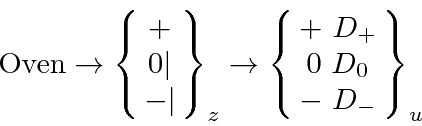We put a detector in each of the beams split into determine the intensity.

To solve this with the rotation matrices, we first determine the state after the first apparatus. It is just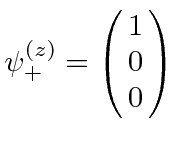with the usual basis. Now we rotate to a new (primed) set of basis states with thealong thedirection. This means a rotation through an angleabout the y direction. The problem didn't clearly define whether it is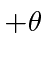or, but, if we only need to know the intensities, it doesn't matter. So the state coming out of the second apparatus isThe 3 amplitudes in this vector just need to be (absolute) squared to get the 3 intensities.These add up to 1.

An alternate solution would be to use theoperator. Find the eigenvectors of this operator, like. The intensity in the + beam is then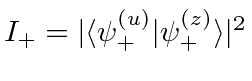.

Jim Branson 2013-04-22